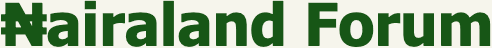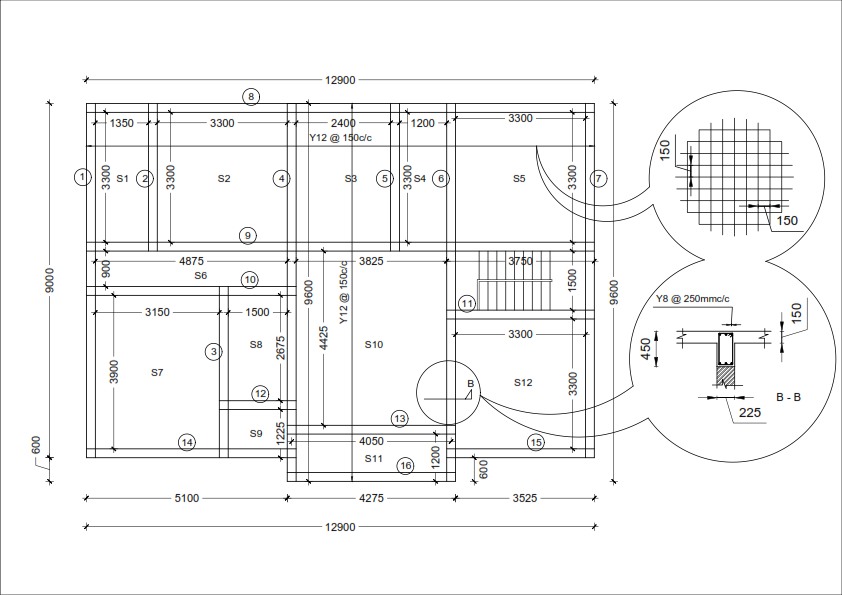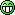#Welcome, Guest: Join Nairaland / LOGIN! / Trending / Recent / New
Stats: 2,595,392 members, 6,020,945 topics. Date: Saturday, 28 November 2020 at 03:03 PM

## How To Calculate Materials Needed For Beam And Slab (decking) - Diy - Properties - Nairaland

 How To Calculate Materials Needed For Beam And Slab (decking) - Diy by saydfact(m): 10:03am On Sep 05 Good day all,So, I got a few 'likes' in the property sections to do this; designed for site supervisors, carpenters and welder/iron benders, contractors and DIY aspiring home owners. A lot of people have lost job opportunity because they 'over quoted' and others have fallen victim to under-quoting resulting in them running-away or abandoning the projects.We would practically calculate all the materials need for the below 3 bedroom apartment (plan attached) - at the end of this tutorial, I expect that your estimation of beams and slab (decking) should be better or closer to reality.Let's get to business - attached below is the slab (S1,S2,S3, etc) and beam (1,2,3, etc) design.Let’s get to business; below is a list of items we would be calculating.1. Bamboo2. 2by3 wood3. 1x12 plank4. 8/10mm rings in Beam5. 16mm bars in Beam6. 12mm bars in slab7. Granite, Sand and Cement in beam & slab8. Estimates for others (binding wires, nails etc)To get a more accurate result, I have divided the slab into sections (S1, S2, S3 etc) - it is possible to take all the slabs as 1 unit for more experienced persons. (the method we would use is give detailed calculation for 2 or 3 sections and state the answer for other (to safe thread space).NOTE: All dimensions are in Millimetre *1. BAMBOBamboo are placed at 600mm c/c (center-to-center), and are placed in orderly manner vertically and horizontally - hence all we need calculate is number along the X axis and along the Y axis and multiple for numbers. . . . lets go practical now.SLAB S1 = 1350 by 3300. Number of Bamboo along any axis = (Distance / 600) + 1 hence: (1350 / 600 ) + 1 = 3 and (3300 / 600) + 1 = 6number of Bamboos needed for Slab S1 = 3 X 6 = 18 nosSLAB S2 = 3300 by 3300.>> (3300 / 600 ) +1 and (3300 / 600) + 1>> 6 x 6 = 36 nosSLAB S3 = 2400 by 3300.>> (2400 / 600 )+1 and (3300 / 600)+1>> 5 x 6 = 30 nosNow; calculate for SLAB S4 to S12 S4 = 21 nos; S5 = 36 nos; S6 = 18 nos; S7 = 48 nos; S8 = 15 nos; S9 = 9 nos; S10 = 56 nos; S11 = 21 nos and S12 = 36 nosTotal nos = 344 nos (BUT this is not the total number of Bamboo to buy EXCEPT you're buying used bamboos)Because New bamboos come in about 10m up; divide the answer by 2 = 344 / 2 = 172 pieces (the off-cuts would take care of minor bracing)Bamboo for temporal (mallam) staircase used for casting = approx number = 10 pieces.TOTAL BAMBOO TO BUY = 172 + 10 = 185 PIECES (Approx to nearest /5)2. 2by3 WOOD2x3 woods are placed directly on the bamboo and under the 1by12 planks, to transmit the load across and hold all the plank in place. It is also placed at 600mm c/c and has almost same method of calculation as the bamboo. (for ease, we would also calculate it in sections)Lets calculate the quantity of 2x3 needed for S1 (pick any side to align the wood with; I'll pick the 1350mm side - hence its the 3300 that i'll divide with 600)>> (3300 / 600 )+1 >> 7. (THIS MEANS, I need 7 number of 1350mm wood) >> 1350 x 7 = 9450mm (I need total length of 9450mm wood & each wood is going to be probably 3000mm long >> this means I need 9450 / 3000 >> 3 length of 2x3 wood for slab S1.Lets calculate S1 again picking 3300 as our alignment - hence we divide (1350/600) + 1 = 3>> 3300 x 3 = 9900mm (total length of wood needed)if wood is 3000mm long, then we need 9900 / 3000 >> 3 length of 2x3 wood for slab S1.1 x 3 = 3 lengths needed.ALWAYS APPROXIMATE TO THE NEAREST WHOLE NUMBER.LETS calculate S2 by quicker now.3300 by 3300, we align to the first 3300 and divide the second 3300mm by 600mm spacing.>> (3300 / 600) +1 = 7>> 3300 x 7 (the 2nd 3300 was used) = 23100mm of wood needed>> 23100 / 3000 = 8 lenght of 2x3 wood for slab S2.SLAB S3>> (3300 / 600) +1 = 7>> 2400 x 7 = 16800mm of wood needed>> 16800 / 3000 = 6 length of 2x3 wood for slab S3.Now; calculate for SLAB S4 to S12S4 = 3nos, S5 = 8 nos, S6 = 3 nos, S7 = 8 nos, S8 = 3 nos, S9 = 2, S10 = 10, S11 = 4, S12 = 8 (Total = 49 nos)OTHERS (2x3 wood)There are additional usage for 2x3 wood, in the mallam steps = 10 (approx)and cut into sizes of 225mm (used to hold the beam in place) = 10 (approx)in Staircase = 5 (approx)TOTAL 2 x 3 WOOD TO BUY = 91 nos >>> BUY 95 PIECES3. 1 by 12 PLANKA. FOR BEAM:Estimating for beams isn't that difficult, all we need to do is get the TOTAL LINEAR LENGTH of the beams (additions of all the lengths of beam 1 to beam 16) AND divide by the length of 1 plank (3000mm).So; Beam1 = 9000, Beam2 = 3300, Beam3 = 3900, B4 = 9600, B5 = 3300, B6 = 9600, B7 = 9000, B8 = 12900, B9 = 12900, B10 = 4875, B11 = 3300, B12 = 1500, B13 = 4050, B14 = 5100, B15 = 3525, B16 = 4275.TOTAL LINEAR LENGTH OF BEAM = 100125mm (saved this length, we would use it again soon)Number of planks needed to cover 1 side of the beam >> 100125/3000 (length of 1 plank) >> 33 planks (BUT THERE ARE 2 SIDES TO A BEAM)Hence; 1x12 planks needed for beam = 66 nos (we would add for waste later)B. FOR SLAB:The fastest way to calculate for slab is to find the area of the slab and divide by the area of 1 plank OR you can calculate for each Slab 1 to 12). I'LL SO AN EXAMPLE OF THE SLAB1 -12, then use the TOTAL AREA option.E.G Area of 1 Plank = 3000 x 300 (LxB) = 900,000mmArea of Slab S1 = 3300 x 1350 = 4,455,000Number of 1x12 plank = 4,455,000 / 900,000= 5 PlanksSlab S2 (another Example)Area of Slab S2 = 3300 x 3300 = 10,890,000mmArea of plank = 900,000mmNumber of 1x12 planks = 10,890,000/900,000= 12 PlanksI'm going to save time and disregard the two examples above for a shorter method (DISREGARD S1 and S2 ABOVE)PLANKS NEEDED FOR SLAB:TOTAL AREA OF SLAB / AREA OF 1 PLANKTotal area of slab = (12900 x 9000 ) + (600 x 4275) >> 116,100,000 + 2,565,000 = 118,665,000Hence >> 118,665,000 / 900000 = 132 planks (we would add for waste later)C. FOR EDGES OF SLAB:In the case of side to slab (150mm thick except otherwise stated in the structural drawings). The plank is sawed (cut) to size at the sawmill; this helps the bricklayers when casting the floor and acts as a level. You could also use as it is BUT you would be required to use a nail to mark out the 150mm level.Since the plank is used along the PERIMETER of the slab, all we need do is add all the sides of the slab to get the perimeter.from our plan; Perimeter = 12900 + 9000 + 3525 + 600 + 4275 + 600 + 5100 + 9000 = 45000mm i.e how many planks of 3000mm long would be required to fill the above length ?>> 45000 / 3000 = 15 nos. Its advisable to add 1 to it because its sliced to size and better than repeating the process.Total planks needed = 16 planks (waste added for already)D. FOR STAIRS AND TEMPORAL (MALLAM) STAIRS:Lets assume our stairs is 900mm wide (thats the size of 3 planks - note that if its 1200 wide. its 4 planks side by side)The average staircase measures about 6000mm long (minus landing) hence:>>> 3 planks side by side and 2 planks long = 6 planks + about 4 planks for landing and side, should do the job.Hence = 10 planksfor Mallam stairs, the difference is much EXCEPT thats would be wider than 900mm, minimum is 1200mm, hence:>> 4 planks side by side and 3 planks long = 12 planks + about 2 for landing should doHence = 14 planksTOTAL PLANKS NEEDED ON THIS PROJECT = 66 + 132 + 16 + 10 + 14 = 238 nosDon't be an Ijebu-Man like me; CONSIDER WASTE and BUY >> 250 PIECES4. 8mm OR 10mm IN RINGSFrom the design our beam is 450mm x 225mm HENCE our ring would be 450-50mm x 225-50mm (where 50 is gotten from the concrete cover of 25mm on all the four sides of the ring. (OUR RING = 400mm by 175mm).Calculate the perimeter of the ring = 400 + 175 + 400 + 175 = 1150 (add 100mm for overlap of rings) >> 1250mm long.What this means is that, to produce 1 ring, I need 8mm or 10mm rod of length 1250mm. (BINGO)The design also used ring spacing at 250mm (or as designed by the structural engineer).REMEMBER OUR TOTAL LINEAR LENGTH OF BEAM IS = 100125mmHence >> 100125 mm / 250mm (spacing) gives us the total number of rings needed.>> 401 rings are needed but ONE ring is 1250mm longHence: 1 lenght of Iron (12000mm) long can produce 9 rings. (i.e 12000 / 1250)IF 1 IRON ROD PRODUCES 9 RINGS, HOW MANY RODS IS NEEDED TO PRODUCE 401 RINGS ?Ans = 401 / 9 >> BUY 45 LENGTH OF 8mm/10mm5. 16mm BARS IN BEAMAlso from our design (except otherwise designed), we have 4Y16 = 2 bars of 16mm top and 2 bars of 16mm bottom = 4.LINEAR LENGTH OF BEAM = 100125mm1 LENGTH OF 16mm ROD -= 12000mmWe need 100125 / 12000 to fill the entire length >> 9 Bars there are 4 bars to fill, hence we need 9 x 4 = 36. BUT DEY OOOHHHHThis particular bar has a lot of overlap, so we would allow 20% for overlap = 6 bars.TOTAL NOS OF 16mm NEEDED >> BUY 42 PIECES 6. 12mm IN SLABCalculating 12mm in slab, need a little technicality, you need to have an idea of how the work is done, but here is a simple task you can always do.A. Find the width of the slab (in this case, its 9600mm), add 1000mm for return on both sides (1000 x 2 = 2000). IF THE ANSWER YOU GET IS MORE THAN 12000mm (YOU'LL NEED TO ADD ANOTHER 1200mm for overlap (i.e 600 x 2 ) where 60mm is sufficient overlap for 12mm rebars.In our slab >> 9600 + 2000 = 11600mm (so we dont need to add for overlap because 1 LENGTH of 12mm rebar would complete 1 line.From the design; our bars are spaced at 150mm C/C (centre to centre), Hence >> How many lines of 150mm would fill the LENGHT (12900mm) of the slab ?>> 12900 / 150 = 86 lines of rebar is needed. We established earlier that 1 rebar would do 1 line hence: 86 x 1 = 86 rebars is needed along the width.Rebars needed along the legth >> 12900 + 2000 + 1200 = 16100mm (i.e Length of slab + return + overlap because the 1st 2 added is more than 12000mm)What the above means is that we need a bar of 16100 for 1 line = 16100/12000 = we need 1.5 bars per line (WE NEED ONE AND HALF LENGTH PER LINES)Number of lines = 9600 / 150 = 64 lines >> 1.5 length x 64 lines = 96 rebars needed along the length.TOTAL REBAR NEEDED FOR SLAB = 86 +96 = 182 LENGTHS.Add another 10 bars for staircases and waste >> BUY 192 LENGTH OF 12mmNote: I didn't deduct the VOID around the stair-hall because it covers for the extra double return needed at that point.7. GRANITE, SAND AND CEMENT IN BEAM & SLABI have so many previous tutorial on this already, there is no need occupying space (I'm sure you all agree - if you need the details, kindly refer to this post and apply same principles >> https://www.nairaland.com/5818635/estimating-cost-fencing-6-plotsVolume of Concrete = 18 cubic meters (m3)GRANITE = 45 tonnesSAND = 20 tonnes (if complete, I'll advice 30 tonnes cos it wont be complete)CEMENT IN BEAM & SLAB = 110 bags8. OTHERSBinding Wire BUY 1.5 BUNDLES; it should do the jobNails - 3 bags (various sizes: 2 inches = 1.5 bag, 3 inches = 1 bag & 4 inches = 0.5 bag)Water - Get 4 tanks on standby THANK YOU FOR READING . . . . Check my profile for similar post on other topics.GOODLUCK. 16 Likes 3 SharesRe: How To Calculate Materials Needed For Beam And Slab (decking) - Diy by saydfact(m): 10:04am On Sep 05 You can also read:How To Calculate The Quantity Of Tiles Needed; Selecting Tiles & Types >> https://www.nairaland.com/5420029/how-calculate-quantity-tiles-neededHow To Practically Calculate The Quantity (m2) Of Roofing Sheet Required >> https://www.nairaland.com/4778694/how-practically-calculate-quantity-m2 1 Like 1 Share Re: How To Calculate Materials Needed For Beam And Slab (decking) - Diy by Teewhy2: 10:05am On Sep 05 very detailed, this should help a lot of house owners during estimation. want to ask can't the bamboo be placed at 600 x 750mm C-C or 750 x 750 mm, will it have a negative effect on the bending moment of the slab. Re: How To Calculate Materials Needed For Beam And Slab (decking) - Diy by saydfact(m): 10:22am On Sep 05 Teewhy2:very detailed, this should help a lot of house owners during estimation. want to ask can't the bamboo be placed at 600 x 750mm C-C or 750 x 750 mm, will it have a negative effect on the bending moment of the slab.The challenge of increasing beyond 600mm is the quality of work done - at 600mm you're assured if any bamboo isn't well placed, the 2 beside it can cover for it - going beyond that might be a risk. Re: How To Calculate Materials Needed For Beam And Slab (decking) - Diy by Johntemmy(m): 10:44am On Sep 05 nice job. Re: How To Calculate Materials Needed For Beam And Slab (decking) - Diy by Teewhy2: 12:06pm On Sep 05 saydfact:The challenge of increasing beyond 600mm is the quality of work done - at 600mm you're assured if any bamboo isn't well placed, the 2 beside it can cover for it - going beyond that might be a risk.thanks noted. 1 Like Re: How To Calculate Materials Needed For Beam And Slab (decking) - Diy by Hargbo(m): 2:47pm On Sep 05 Well done sirRe: How To Calculate Materials Needed For Beam And Slab (decking) - Diy by saydfact(m): 5:39pm On Sep 05 Hargbo:Well done sirThanks Re: How To Calculate Materials Needed For Beam And Slab (decking) - Diy by Mayor78: 8:20pm On Sep 06 Very well detailed. Rogue contractors may not like your effort, but keep it up. Real people will appreciate you. 1 Like Re: How To Calculate Materials Needed For Beam And Slab (decking) - Diy by saydfact(m): 11:58am On Sep 07 Mayor78:Very well detailed. Rogue contractors may not like your effort, but keep it up. Real people will appreciate you. THANKS BOSS - who go make am go make am, no be by duping2 Likes